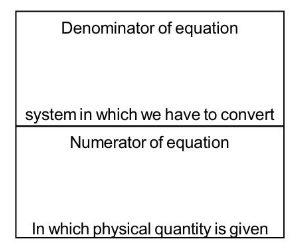Most Affordable JEE | NEET | 8,9,10 Preparation by Kota's Top IITian Doctor Faculties

# What are the uses of Dimensional Equations? and what is Conversion of one system of units into another?## Uses of Dimensional Equations

Following are the uses of dimensional equations.

1. Conversion of one system of units into another

2. Checking the accuracy of various formulas or equation

3. Derivation of Formula.

## Conversion of one system of units into another

Let the numerical values are $n_{1}$ and $n_{2}$ of a given quantity $Q$ in two-unit system and the units are-

$U_{1}=M_{1}^{a} L_{1}^{b} T_{1}^{c}$ and $U_{2}=M_{2}^{a} L_{2}^{b} T_{2}^{c}$

Therefore, By the principle $\mathrm{nu}=$ constant

$\Rightarrow \mathrm{n}_{2} \mathrm{U}_{2}=\mathrm{n}_{1} \mathrm{u}_{1}$

$\mathrm{n}_{2}\left[\mathrm{M}_{2}^{a} \mathrm{~L}_{2}^{b} \mathrm{~T}_{2}^{c}\right]=\mathrm{n}_{1}\left[\mathrm{M}_{1}^{a} L_{1}^{b} \mathrm{~T}_{1}^{c}\right]$

$\Rightarrow \quad \mathrm{n}_{2}=\frac{\mathrm{n}_{1}\left[\mathrm{M}_{1}^{\mathrm{a}} \mathrm{L}_{1}^{\mathrm{b}} \mathrm{T}_{1}^{\mathrm{c}}\right]}{\left[\mathrm{M}_{2}^{\mathrm{a}} \mathrm{L}_{2}^{\mathrm{b}} \mathrm{T}_{2}^{\mathrm{c}}\right]}$

$\Rightarrow \quad n_{2}=\left[\frac{M_{1}}{M_{2}}\right]^{a}\left[\frac{L_{1}}{L_{2}}\right]^{b}\left[\frac{T_{1}}{T_{2}}\right]^{c} n_{1}$Principle of Homogeneity

The dimensions of both sides in an equation are the same.

Ex. $\mathrm{s}=\mathrm{ut}+\frac{1}{2} \mathrm{gt}^{2}$

$[L]=\left[L T^{-1} \cdot T\right]+\left[L T^{-2} \cdot T^{2}\right]$

$[\mathrm{L}]=[\mathrm{L}]+[\mathrm{L}]$